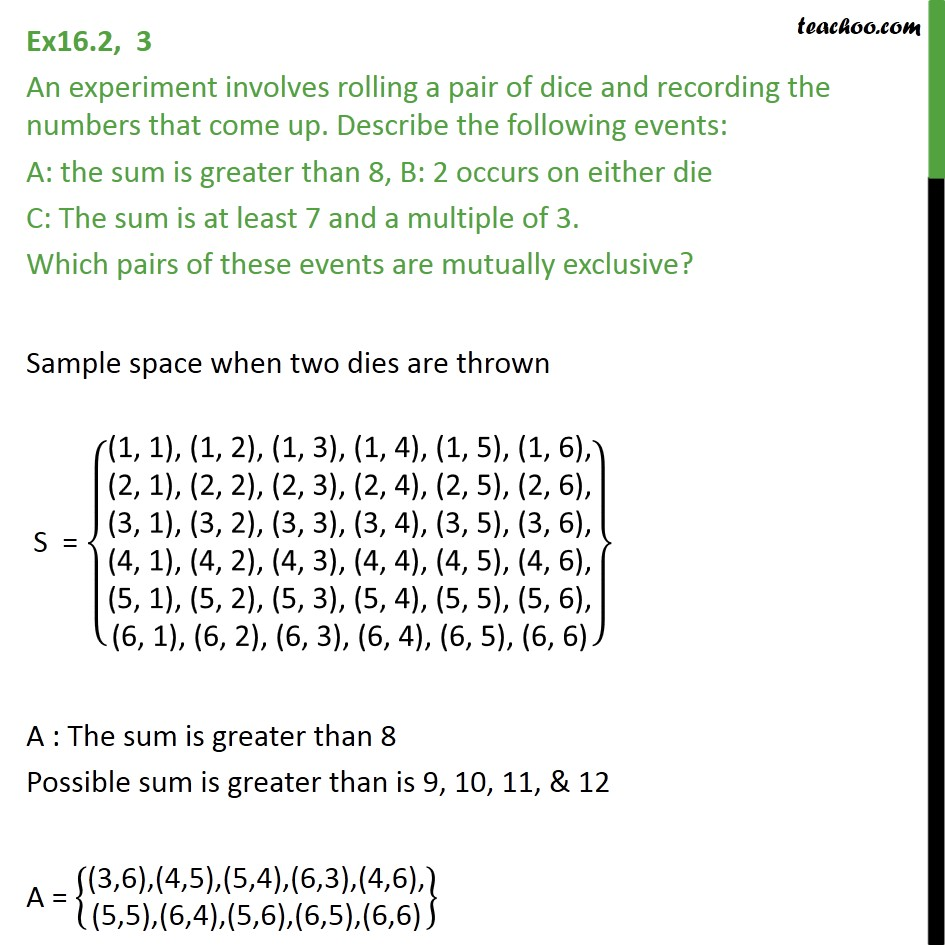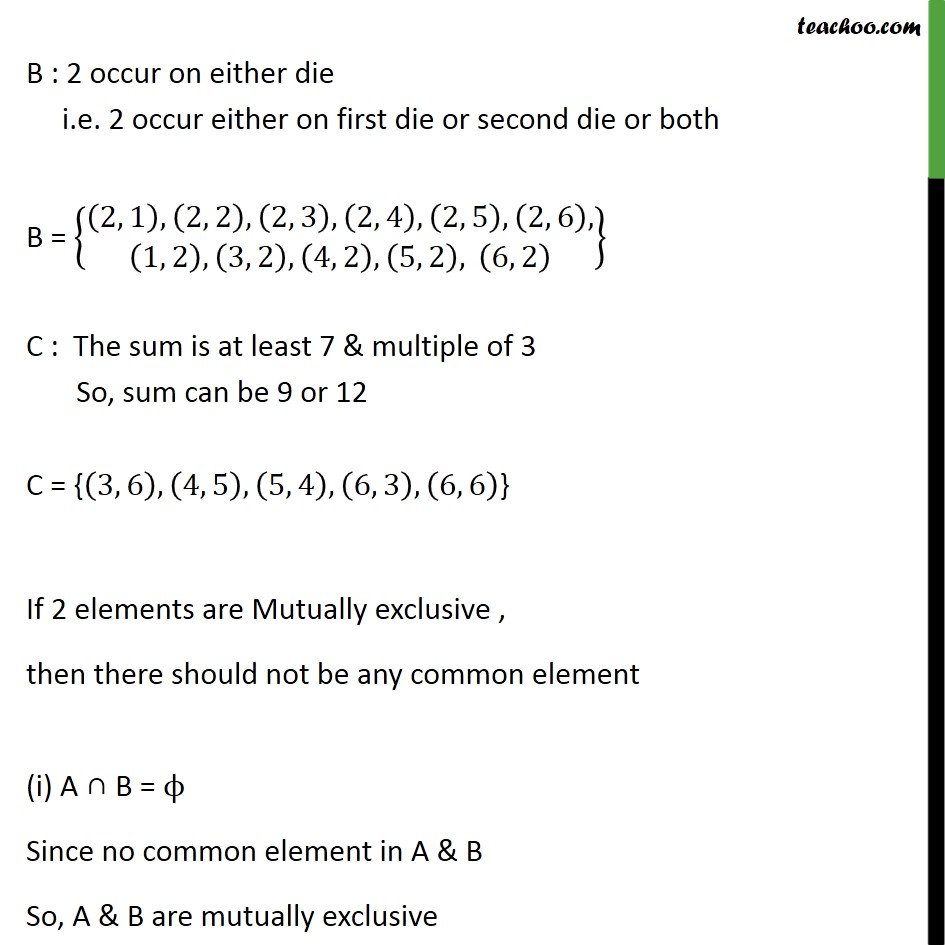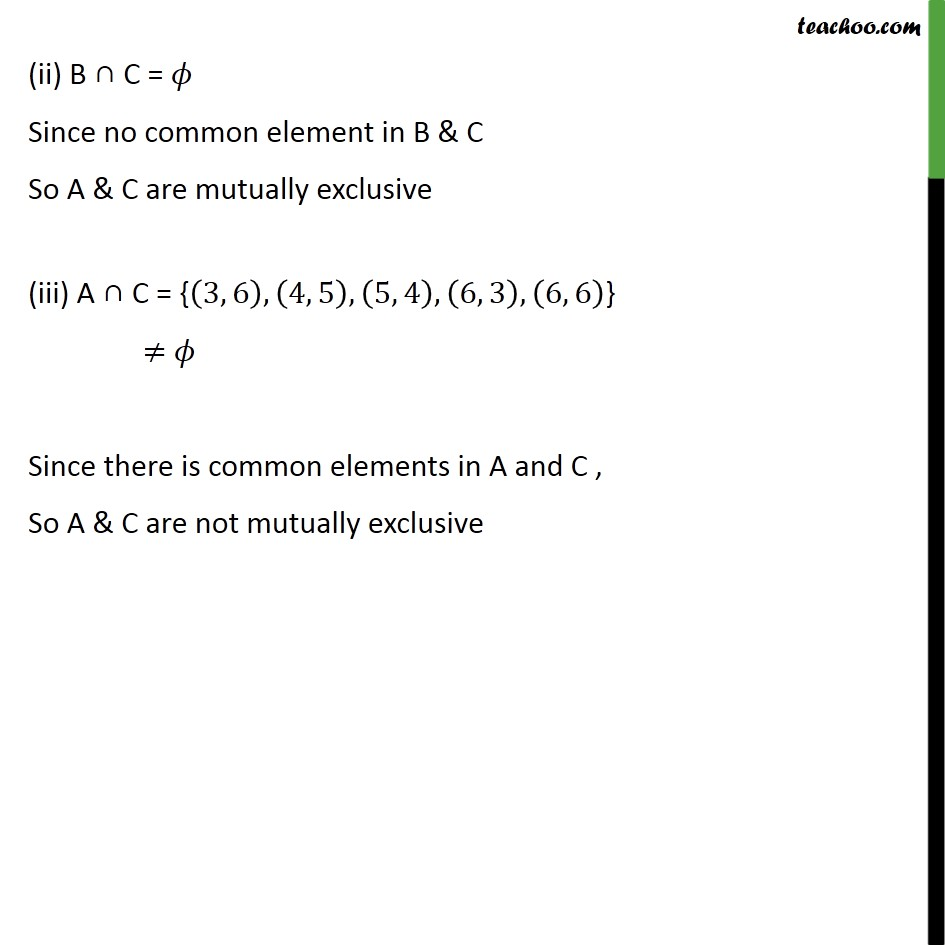Mutually Exculsive and Exhaustive Events

Chapter 14 Class 11 Probability
Concept wiseLearn in your speed, with individual attention - Teachoo Maths 1-on-1 Class

### Transcript

Ex16.2, 3 An experiment involves rolling a pair of dice and recording the numbers that come up. Describe the following events: A: the sum is greater than 8, B: 2 occurs on either die C: The sum is at least 7 and a multiple of 3. Which pairs of these events are mutually exclusive? Sample space when two dies are thrown S = (1, 1), (1, 2), (1, 3), (1, 4), (1, 5), (1, 6),﷮(2, 1), (2, 2), (2, 3), (2, 4), (2, 5), (2, 6),﷮(3, 1), (3, 2), (3, 3), (3, 4), (3, 5), (3, 6),﷮(4, 1), (4, 2), (4, 3), (4, 4), (4, 5), (4, 6),﷮(5, 1), (5, 2), (5, 3), (5, 4), (5, 5), (5, 6),﷮(6, 1), (6, 2), (6, 3), (6, 4), (6, 5), (6, 6)﷯﷯ A : The sum is greater than 8 Possible sum is greater than is 9, 10, 11, & 12 A = (3,6),(4,5),(5,4),(6,3),(4,6),﷮(5,5),(6,4),(5,6),(6,5),(6,6)﷯﷯ B : 2 occur on either die i.e. 2 occur either on first die or second die or both B = 2, 1﷯, 2, 2﷯, 2, 3﷯, 2, 4﷯, 2, 5﷯, 2, 6﷯,﷮ 1, 2﷯, 3, 2﷯, 4, 2﷯, 5, 2﷯, 6, 2﷯﷯﷯ C : The sum is at least 7 & multiple of 3 So, sum can be 9 or 12 C = { 3, 6﷯, 4, 5﷯, 5, 4﷯, 6, 3﷯, 6, 6﷯} If 2 elements are Mutually exclusive , then there should not be any common element (i) A ∩ B = ϕ Since no common element in A & B So, A & B are mutually exclusive (ii) B ∩ C = 𝜙 Since no common element in B & C So A & C are mutually exclusive (iii) A ∩ C = { 3, 6﷯, 4, 5﷯, 5, 4﷯, 6, 3﷯, 6, 6﷯} ≠ 𝜙 Since there is common elements in A and C , So A & C are not mutually exclusive